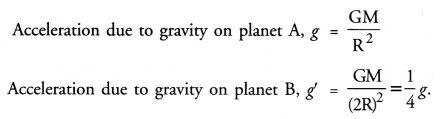## HOTS Questions for Class 9 Science Chapter 10 Gravitation

These Solutions are part of HOTS Questions for Class 9 Science. Here we have given HOTS Questions for Class 9 Science Chapter 10 Gravitation

Question 1.
Gravitational force between two masses m1 and m2 separated by a distance r in air is F. What is the gravitational force between these masses separated by the same distance r in vacuum ?
Gravitational force between two masses does not depend on the medium in which they are placed. Therefore, gravitational force between the masses in vacuum will also be F.

More Resources

Question 2.
Can a body has mass, but no weight ? Give reason for your answer. (CBSE 2015)